Proving Your Americanness: Historical and Contemporary Voter Suppression
State of Louisiana Literacy Test (This test was given during the late 1950s to anyone who was not able to prove that they had a fifth grade education.)

Voter tests were required as a tactic for voter suppression. Each person was given 10 minutes to complete this 30 question test. One wrong answer resulted in the inability to vote. Can you pass this test in 10 minutes?
1. Draw a line around the number or letter of this sentence. *
1 point
2. Draw a line under the last word in this line. *
1 point
3. Cross out the longest word in this line. *
1 point
4. Draw a line around the shortest word in this line. *
1 point
5. Circle the first, first letter of the alphabet in this line. *
1 point
6. In the space below draw three circles, one inside (engulfed by) the other. *
Choose which you think is correct.
1 point
7. Above the letter X make a small cross. *
Choose which you think is correct.
1 point
8. Draw a line through the letter below that comes earliest in the alphabet. *
Choose which you think is correct.
1 point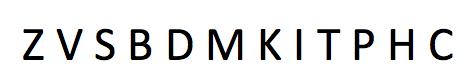9. Draw a line through the two letters below that come last in the alphabet. *
Choose which you think is correct.
1 point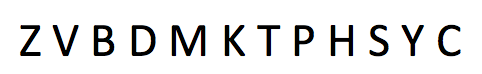10. In the first circle below write the last letter of the first word beginning with "L". *
Choose which you think is correct.
1 point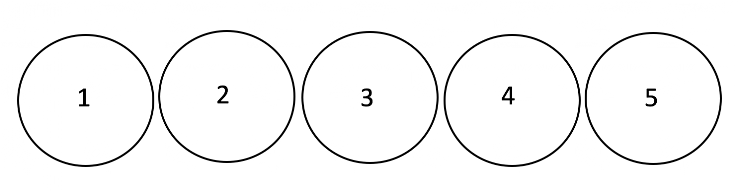11. Cross out the number necessary, when making the number below one million. *
Choose which you think is correct.
1 point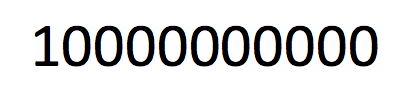12. Draw a line from circle 2 to circle 5 that will pass below circle 2 and above circle 4. *
Choose which you think is correct.
1 point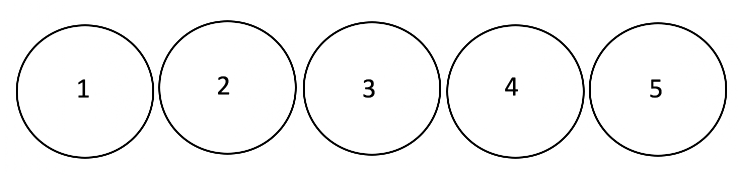13. In the line below cross out each number that is more than 20 but less than 30. *
Choose which you think is correct.
1 point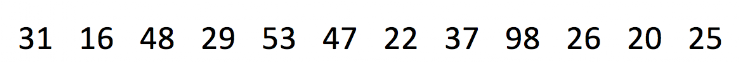14. Draw a line under the first letter after "h" and draw a line through the second letter after "j". *
Choose which you think is correct.
1 point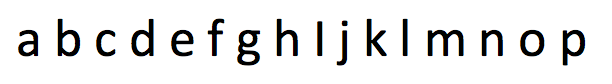15. In the space below, write the word "noise" backwards and state what would be its second letter should it have been written forward. *
1 point
16. Draw a triangle with a blackened circle that overlaps only its left corner. *
Choose which you think is correct.
1 point
17. Look at the line of numbers below, and place on the blank, the number that should come next. *
1 point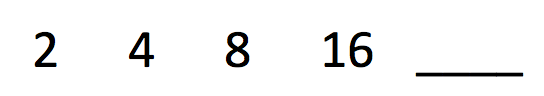18. Look at the line of numbers below, and place on the blank, the number that should come next. *
1 point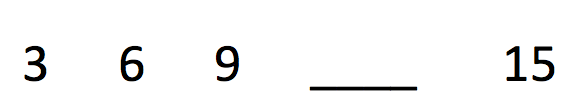19. Draw in the space below, a square with a triangle in it, and within that same triangle draw a circle with a black dot in it. *
Choose which you think is correct.
1 point
20. Spell backwards, forwards. *
1 point
21. Print the word vote upside down, but in the correct order. *
Choose which you think is correct.
1 point
22. Place a cross over the tenth letter in this line, a line under the first space in this sentence, and circle around the last the in the second line of this sentence. *
Choose which you think is correct.
1 point
23. Draw a figure that is square in shape. Divide it in half by drawing a straight line from its northeast corner to its southwest corner, and then divide it once more by drawing a broken line from the middle of its western side to the middle of its eastern side. *
Choose which you think is correct.
1 point
24. Print a word that looks the same whether it is printed frontwards or backwards. *
1 point
25. Write down on the line provided, what you read in the triangle below. *
Write the answer in the short text response.
1 point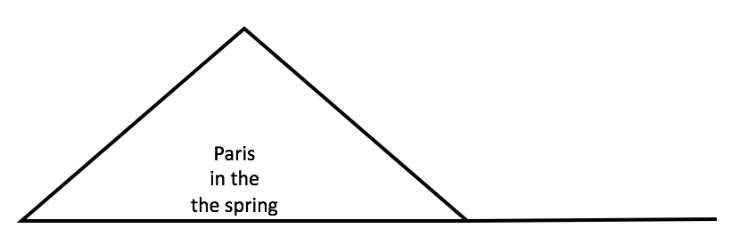26. In the third square below, write the second letter of the fourth word. *
Write the letter.
1 point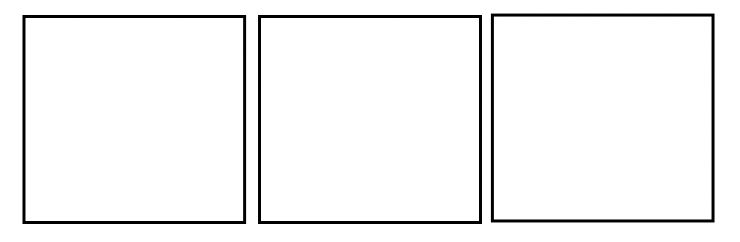27. Write right from the left to the right as you see it spelled here. *
1 point
28. Divide a vertical line in two equal parts by bisecting it with a curved horizontal line that is only straight at its spot bisection of the vertical. *
1 point
29. Write every other word in this first line and print every third word in the same line (original type smaller and first line ended at comma) but capitalize the fifth word that you write. *
1 point
30. Draw five circles that one common inter-locking part. *
Describe
1 point